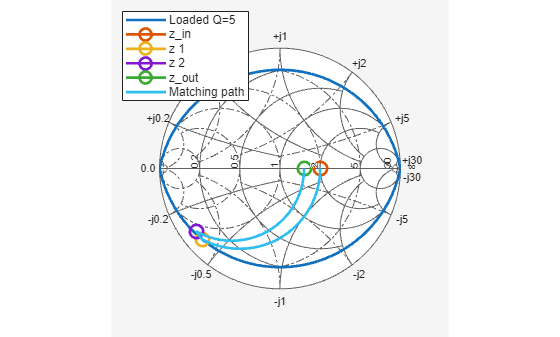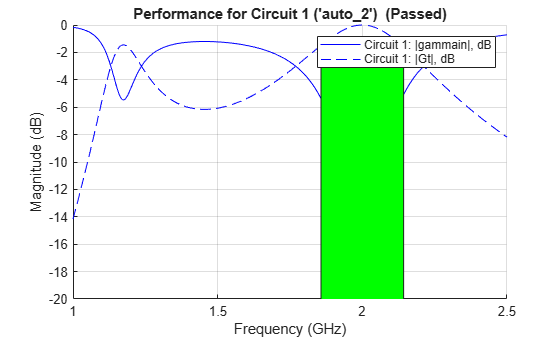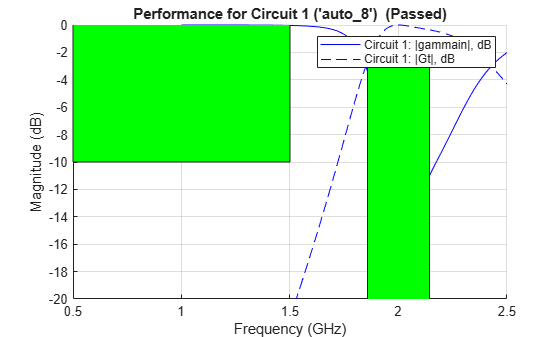matchingnetwork

Create matching network for 1-port network and generate circuit object

Description

Use the matchingnetwork object to create a matching network circuit for a 1-port network which match the impedance of given source to the impedance of given load at a specified center frequency. The matchingnetwork object stores the generated network as a circuit object in the Circuit property. The function exportCircuits could be also used to export the selected circuit(s) generated.

You can use Matching Network Designer app to design, visualize, and compare matching networks for one-port load. For more information, see Matching Network Designer.

Creation

Description

example

matchnet = matchingnetwork creates a matching network object with default property values.

example

matchnet = matchingnetwork(Name,Value) sets properties using one or more name-value pairs. For example, matchnet = matchingnetwork('SourceImpedance','60') creates a matching network with a source impedance of 60 ohms.

Properties

expand all

Source impedance as seen at the terminals looking from the network into the source, specified as one of the following:

• Constant complex scalar in ohms

• sparameters object

• yparameters object

• zparameters object

• File name of a Touchstone file

• One-port circuit object

• Antenna Toolbox™ antenna object

• Function handle to a function that computes an impedance list from a frequency list

Example: 'SourceImpedance',60

Example: matchnet.SourceImpedance = 60

Example: 'SourceImpedance','default.s1p'

Data Types: double | char | string | function_handle

Load impedance as seen at the terminals looking from the matching network into the load, specified as one of the following:

• Constant complex scalar in ohms

• sparameters object

• yparameters object

• zparameters object

• File name of a Touchstone file

• One-port circuit object

• Antenna Toolbox antenna object

• Function handle to a function that computes an impedance list from a frequency list

Data Types: double | char | string | function_handle

Frequency to calculate the impedance match between the source and the load, specified as a real positive scalar in hertz

Example: 'CenterFrequency',1e9

Example: matchnet.CenterFrequency = 1e9

Data Types: double

Desired bandwidth (transducer gain >= minus 3 dB over this bandwidth centered on CenterFrequency), specified as a real positive scalar in hertz.

Example: 'BandWidth',100e6

Example: matchnet.BandWidth = 100e6

Data Types: double

Desired loaded quality factor, specified as a real positive scalar. Setting LoadedQ updates the bandwidth. If you specify CenterFrequency, LoadedQ is recalculated from CenterFrequency and BandWidth.

Data Types: double

Note

Number of components or type of topology for the matching network design, specified as 2 or 3 for the number of components and 'Pi', 'Tee', or, 'L' for the type of topology.

Example: 'Components','Pi'

Example: matchnet.Components = 'Pi'

Data Types: double | char | string

An array of circuit objects containing possible matching network designs for the given set of parameters.

Note

Object Functions

 addEvaluationParameter Adds performance goal for sort, pass, or fail matching network design circuitDescriptions Tables describing each created matching network's topology and performance getEvaluationParameters Table of evaluation parameters currently used to rank and pass or fail matching network designs clearEvaluationParameter Delete one or more performance goals exportCircuits Select and export generated matching networks as circuit objects from an existing matching network object rfplot Plot input reflection coefficient and transducer gain of matching network smithplot Plot measurement data on Smith chart sparameters Calculate S-parameters for RF data, network, circuit, and matching network objects

Examples

collapse all

Create a default matching network using the object, matchingnetwork.

matchnet = matchingnetwork
matchnet =
matchingnetwork with properties:

SourceImpedance: 50 Ohms
CenterFrequency: 1 GHz
Components: 2
Circuit: [1x2 circuit]

Create a matching network with source impedance, 100 ohms, load impedance, 75 ohms, center frequency, 2 GHz, desired loaded quality factor, 5, and the number of components, 3.

mnobj =
matchingnetwork with properties:

SourceImpedance: 100 Ohms
CenterFrequency: 2 GHz
Bandwidth: 400 MHz
Components: 3
Circuit: [1x8 circuit]

Display the list of matching network circuits generated and their corresponding performance

[circuit_list, performance] = circuitDescriptions(mnobj)
circuit_list=8×7 table
circuitName    component1Type    component1Value    component2Type    component2Value    component3Type    component3Value
___________    ______________    _______________    ______________    _______________    ______________    _______________

Circuit 1     "auto_2"        "Shunt C"         3.9789e-12         "Series L"        2.1389e-10         "Shunt L"         1.3876e-09
Circuit 2     "auto_7"        "Series C"        1.8501e-13         "Shunt C"         2.8519e-14         "Series L"        2.9842e-08
Circuit 3     "auto_3"        "Shunt L"         1.5915e-09         "Series C"        2.9607e-11         "Shunt C"         4.5637e-12
Circuit 4     "auto_6"        "Series L"        3.4228e-08         "Shunt L"         2.2205e-07         "Series C"        2.1221e-13
Circuit 5     "auto_1"        "Shunt C"         3.9789e-12         "Series L"        2.8468e-09         "Shunt C"         4.5637e-12
Circuit 6     "auto_5"        "Series L"        3.4228e-08         "Shunt C"         3.7957e-13         "Series L"        2.9842e-08
Circuit 7     "auto_4"        "Shunt L"         1.5915e-09         "Series C"        2.2245e-12         "Shunt L"         1.3876e-09
Circuit 8     "auto_8"        "Series C"        1.8501e-13         "Shunt L"         1.6684e-08         "Series C"        2.1221e-13

performance=8×4 table
circuitName    evaluationPassed    testsFailed     performanceScore
___________    ________________    ____________    ________________

Circuit 1     "auto_2"         {["Yes"]}        {0x0 double}      {[ 1.9447]}
Circuit 2     "auto_7"         {["Yes"]}        {0x0 double}      {[ 1.9447]}
Circuit 3     "auto_3"         {["Yes"]}        {0x0 double}      {[ 1.9443]}
Circuit 4     "auto_6"         {["Yes"]}        {0x0 double}      {[ 1.9443]}
Circuit 5     "auto_1"         {["No" ]}        {[       1]}      {[-0.1254]}
Circuit 6     "auto_5"         {["No" ]}        {[       1]}      {[-0.1254]}
Circuit 7     "auto_4"         {["No" ]}        {[       1]}      {[-0.6947]}
Circuit 8     "auto_8"         {["No" ]}        {[       1]}      {[-0.6947]}

Plot the frequency response of the best circuit (Circuit #1) between 0.5 GHz and 2.5 GHz.

frequencies     = linspace(0.5e9,2.4e9);
CircuitIndex    = 1; % Best circuit is sorted to the top
rfplot(mnobj,frequencies,CircuitIndex)Plot impedance transformation for the best matching network generated (Circuit#1). For more information, see smithplot.

smithplot(mnobj)To export a selected matching network circuit, for example, Circuit #5:

CircuitIndex    = 5;
mn_circuit      = mnobj.Circuit(CircuitIndex)
mn_circuit =
circuit: Circuit element

ElementNames: {'C'  'L'  'C_1'}
Elements: [1x3 rf.internal.circuit.RLC]
Nodes: [1 2 3]
Name: 'auto_1'
NumPorts: 2
Terminals: {'p1+'  'p2+'  'p1-'  'p2-'}

Alternatively, use exportCircuits(m,CircuitIndex).

Show the default evaluation parameters used by the matching network.

ep = getEvaluationParameters(mnobj)
ep=1×6 table
Parameter    Comparison     Goal               Band               Weight       Source
_________    __________    ______    _________________________    ______    _____________

{'Gt'}        {'>'}       {[-3]}    {[1.8000e+09 2.2000e+09]}    {}     {'Automatic'}

Add a new evaluation parameter and plot the frequency response of Circuit #1.

mnobj =
matchingnetwork with properties:

SourceImpedance: 100 Ohms
CenterFrequency: 2 GHz
Bandwidth: 400 MHz
Components: 3
Circuit: [1x8 circuit]
rfplot(mnobj,frequencies,1)Create a dipole antenna and create the S-parameters of the antenna. This example requires Antenna Toolbox.

d       = dipole('Length', 0.103, 'Width',0.0022);
freq    = linspace(0.5e9,2.5e9,1001);
sd      = sparameters(d, freq);

Alternatively, load S-Parameters from the MAT file

Create a matching network from the S-parameters.

Get the evaluation parameters of the network.

t = getEvaluationParameters(n)
t=1×6 table
Parameter    Comparison     Goal               Band               Weight       Source
_________    __________    ______    _________________________    ______    _____________

{'Gt'}        {'>'}       {[-3]}    {[1.8571e+09 2.1429e+09]}    {}     {'Automatic'}

Plot the reflection coefficient and transducer gain of the matching network circuit 1 , at a frequency range of 1 GHz to 2.5 GHz.

rfplot(n, (1e9:0.001e9:2.5e9),1);Add a new evaluation parameter to compare the transducer gain to have a cut-off of less than -10 dB. Use a frequency range of 0.5 GHz to 1.5 GHz. Plot the comparisons.

n = addEvaluationParameter(n, 'Gt', '<', -10, [0.5e9 1.5e9], 1);
t = getEvaluationParameters(n)
t=2×6 table
Parameter    Comparison     Goal                Band               Weight          Source
_________    __________    _______    _________________________    ______    __________________

{'Gt'}        {'>'}       {[ -3]}    {[1.8571e+09 2.1429e+09]}    {}     {'Automatic'     }
{'Gt'}        {'<'}       {[-10]}    {[ 500000000 1.5000e+09]}    {}     {'User-specified'}

rfplot(n, (1e9:0.001e9:2.5e9),1);Clear evaluation parameters.

n = clearEvaluationParameter(n,1);
t = getEvaluationParameters(n)
t=1×6 table
Parameter    Comparison     Goal                Band              Weight          Source
_________    __________    _______    ________________________    ______    __________________

{'Gt'}        {'<'}       {[-10]}    {[500000000 1.5000e+09]}    {}     {'User-specified'}

This example shows how to calculate the S-Parameters for a newly created matching network for the auto-generated circuit #2 with a reference impedance of 100 Ohm.

freq    = linspace(n.CenterFrequency-n.Bandwidth/2,n.CenterFrequency+n.Bandwidth/2);
RefZ0   = 100;
ckt_no  = 2;
s       = sparameters(n,freq,RefZ0,ckt_no)
s =
sparameters: S-parameters object

NumPorts: 2
Frequencies: [100x1 double]
Parameters: [2x2x100 double]
Impedance: 100

rfparam(obj,i,j) returns S-parameter Sij

 Ludwig, Reinhold, and Gene Bogdanov. RF Circuit Design: Theory and Applications. Prentice-Hall, 2009.

 Bowick, Chris, et al. RF Circuit Design. 2nd ed, 2008.